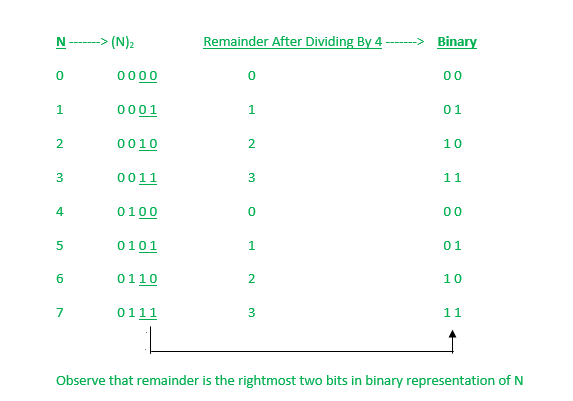# Find the remainder when N is divided by 4 using Bitwise AND operator

Given a number N, the task is to find the remainder when N is divided by 4 using Bitwise AND operator.

Examples:

```Input: N = 98
Output: 2
Explanation:
98 % 4 = 2. Hence the output is 2.

Input: 200
Output: 0
Explanation:
200 % 4 = 0. Hence output is 0.
```

## Recommended: Please try your approach on {IDE} first, before moving on to the solution.

Naive approach:

For solving the above-mentioned problem we can use a naive method by using the Modulo (%) operator to find the remainder. But, the Modulo operator is computationally expensive and the method is inefficient.

Efficient Approach:

If we carefully observe the binary representation of N and its remainder with 4, we observe that remainder is simply the rightmost two bits in N. To get the rightmost two bits in number N, we perform bitwise AND (&) with 3 because 3 in binary is 0011. To understand the approach better let us have a look at the image below:Below is the implementation of the above approach:

## C

 `// C implementation to find N ` `// modulo 4 using Bitwise AND operator ` ` `  `#include ` ` `  `// Function to find the remainder ` `int` `findRemainder(``int` `n) ` `{ ` ` `  `    ``// Bitwise AND with 3 ` `    ``int` `x = n & 3; ` ` `  `    ``// return  x ` `    ``return` `x; ` `} ` ` `  `// Driver code ` `int` `main() ` `{ ` ` `  `    ``int` `N = 43; ` `    ``int` `ans = findRemainder(N); ` ` `  `    ``printf``(``"%d"``, ans); ` ` `  `    ``return` `0; ` `} `

## C++

 `// C++ implementation to find N ` `// modulo 4 using Bitwise AND operator ` ` `  `#include ` `using` `namespace` `std; ` ` `  `// Function to find the remainder ` `int` `findRemainder(``int` `n) ` `{ ` `    ``// Bitwise AND with 3 ` `    ``int` `x = n & 3; ` ` `  `    ``// Return  x ` `    ``return` `x; ` `} ` ` `  `// Driver code ` `int` `main() ` `{ ` ` `  `    ``int` `N = 43; ` ` `  `    ``int` `ans = findRemainder(N); ` ` `  `    ``cout << ans << endl; ` ` `  `    ``return` `0; ` `} `

## Java

 `// Java implementation to find N ` `// modulo 4 using Bitwise AND operator ` ` `  `class` `Main { ` ` `  `    ``// Driver code ` `    ``public` `static` `void` `main(String[] args) ` `    ``{ ` ` `  `        ``int` `N = ``43``; ` ` `  `        ``int` `ans = findRemainder(N); ` ` `  `        ``System.out.println(ans); ` `    ``} ` ` `  `    ``// Function to find the remainder ` `    ``public` `static` `int` `findRemainder(``int` `n) ` `    ``{ ` `        ``// Bitwise AND with 3 ` `        ``int` `x = n & ``3``; ` ` `  `        ``// return  x ` `        ``return` `x; ` `    ``} ` `} `

## Python 3

 `# Python 3 implementation to find N ` `# modulo 4 using Bitwise AND operator ` ` `  `# Function to find the remainder ` `def` `findRemainder(n): ` `    ``# Bitwise AND with 3 ` `    ``x ``=` `n & ``3` ` `  `    ``# Return  x ` `    ``return` `x ` ` `  `# Driver code ` `if` `__name__ ``=``=` `'__main__'``: ` `    ``N ``=` `43` ` `  `    ``ans ``=` `findRemainder(N) ` ` `  `    ``print``(ans) ` `     `  `# This code is contributed by Surendra_Gangwar `

## C#

 `// C# implementation to find N ` `// modulo 4 using Bitwise AND operator ` `using` `System; ` `  `  `class` `GFG { ` `  `  `    ``// Driver code ` `    ``public` `static` `void` `Main() ` `    ``{ ` `  `  `        ``int` `N = 43; ` `  `  `        ``int` `ans = findRemainder(N); ` `  `  `        ``Console.Write(ans); ` `    ``} ` `  `  `    ``// Function to find the remainder ` `    ``public` `static` `int` `findRemainder(``int` `n) ` `    ``{ ` `        ``// Bitwise AND with 3 ` `        ``int` `x = n & 3; ` `  `  `        ``// return  x ` `        ``return` `x; ` `    ``} ` `} ` ` `  `# This code is contributed by chitranayal `

Output:

```3
```

Time Complexity: O(1)

Attention reader! Don’t stop learning now. Get hold of all the important DSA concepts with the DSA Self Paced Course at a student-friendly price and become industry ready.

My Personal Notes arrow_drop_upCheck out this Author's contributed articles.

If you like GeeksforGeeks and would like to contribute, you can also write an article using contribute.geeksforgeeks.org or mail your article to contribute@geeksforgeeks.org. See your article appearing on the GeeksforGeeks main page and help other Geeks.

Please Improve this article if you find anything incorrect by clicking on the "Improve Article" button below.